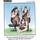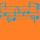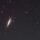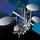## General Question# What is the mathematical constant of "e" mean?

Asked by talljasperman (21858) January 20th, 2015

Can you teach me more about it?

Observing members: 0Composing members: 0Good question! I have wondered this myself, and even after professors explaining it to me, it seems that there’s not one “meaning” for it; it’s just useful. Examples:

1) The exponential function (eΛx) is its own derivative, so it’s very useful for differentiation. f(x)=eΛx also makes the only differential equation such that f(x)=f’(x) [other than perhaps 0].
2) The value e can be used to describe exponential growth (also, the function (1+1/x)^x limits to e as x goes to infinity)
3) It can be described as the sum of the infinite series 1/n! where n starts at 0.
4) It’s the base of the natural logarithm, so eΛ(lnx)=x.

dxs (15079)“Great Answer” (3) Flag as…It doesn’t really “mean” anything. It’s just an irrational number that shows up a lot in nature, and is very useful in physics.

osoraro (2866)“Great Answer” (4) Flag as…@osoraro – it thus follows that the logarthm of e is called Natural Log:
http://en.wikipedia.org/wiki/Natural_logarithm

RocketGuy (11616)“Great Answer” (2) Flag as…Here is a way that the idea of e may have initially arisen. Suppose you put \$1 in bank that is giving 100% interest (not likely, but it makes things simple to work with). How much do you have at the end of a year for a given number of compounding periods, and what is the limit as the number of compouning periods goes to infinity? If the money is compounded n times per year, the effective interest rate in a compound period is 1/n, increasing your principal by a factor of (1+1/n). At the end of the year, this will have been done n times, so you have (1 + 1/n)^n. It may at first seem that if n is taken large enough, you can become a millionaire, but in fact the limit as n goes to infinity is e.

When you express this in terms of calculus, what you are saying is that the instantaneous rate of increase is equal to principal, or dx/dt = x. It is therefore not that surprising that the solution to this equation is a constant times e raised to the t.

LostInParadise (27910)“Great Answer” (1) Flag as…or